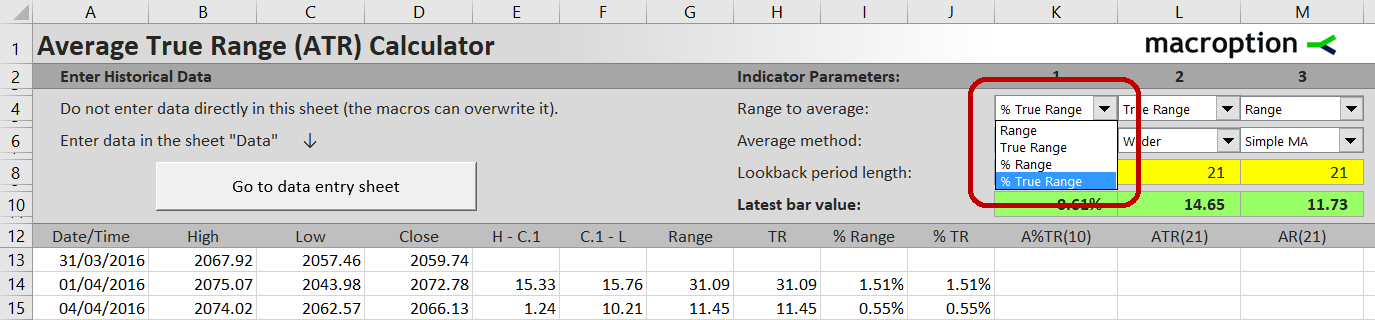# Normalized ATR: Two Ways of Expressing ATR as Percentage

Average True Range (ATR) is a very useful measure of volatility, but it has downsides. Because it is derived from range (or to be precise, true range) and expressed as absolute dollar value, it is not directly comparable across securities and over time.

For example, a stock trading around 10 with ATR of 0.5 is actually more volatile than a stock trading around 200 with a much greater ATR of 2.

## Normalized ATR Logic and Formula

There is an easy fix. If you express ATR as percentage of stock price, you get a volatility measure that is directly comparable across stocks with different prices. In our example, the first stock's ATR becomes 0.5 / 10 = 5% and the second 2 / 200 = 1%. The first stock, which has 4x smaller ATR, is actually 5x more volatile!

This ATR adjustment has a name – it's called Normalized ATR and it was first described by John Forman in a 2006 article titled Cross-Market Evaluations With Normalized Average True Range.

John Forman's formula for Normalized ATR, or NATR, is:

NATR = ATR / Close · 100%

## An Alternative Approach

The good thing about the formula above is that it is very easy to calculate when you already know the ATR.

That said, personally I prefer a slightly different way of normalizing ATR.

John Forman's approach calculates ATR as usual and only then divides the result by the current closing price. The order of operations is: true range – average – normalize.

I prefer to calculate normalized true range first and only then calculate the average of this normalized true range. The order is: true range – normalize – average. Instead of "Normalized Average True Range", I calculate "Average Normalized True Range".

The formula is:

Normalized True Range = Max ( H – L, H – C1, C1 – L ) / C1

Then calculate ATR as usual, only using this normalized true range instead of dollar true range.

Note: In the ATR Calculator, you can calculate this method by selecting "% True Range" instead of "True Range" in the combo in cell K4 / L4 / M4.In my view, normalizing each bar's true range before calculating the average is mathematically cleaner and easier to interpret, as you are working with percentages from the very beginning. It is pure volatility, without any bias from price direction. The other method can overstate volatility in a downtrend and understate it in an uptrend, especially when using a long ATR period – although admittedly, this bias is negligible except for very extreme situations and either of the two versions of Normalized ATR will serve its purpose in most cases.

## When to Use Normalized ATR

Regardless of which of the two Normalized ATR methods you choose, you should use Normalized ATR instead of the traditional, absolute dollar ATR particularly in these situations:

• When using ATR for stock screening (to decide which stocks or securities currently have the right volatility for your strategy or trading style).
• When using ATR as strategy filter (to decide whether the volatility is high enough or low enough to take a signal from a trading strategy, or to "turn on/off" strategies based on market volatility regime).
• When using ATR to study seasonality or volatility patterns over long periods of time.

In general, Normalized ATR is better than traditional ATR in situations which involve comparing different securities or different periods of time.

## When to Use Non-Normalized ATR

On the contrary, the traditional non-normalized ATR is preferred as input in risk management or position management decisions, such as the following:

• Position size (typically calculated as your risk budget / current volatility as ATR)
• Stop loss (typically the distance of stop loss price from entry price or current price is a multiple of ATR)
• Profit target (same logic as stop loss, just the other direction)

In general, traditional non-normalized ATR is better when you are interested in absolute dollar amounts rather than percentages, which is usually when the decision relates to your particular trading capital.

By remaining on this website or using its content, you confirm that you have read and agree with the Terms of Use Agreement.

We are not liable for any damages resulting from using this website. Any information may be inaccurate or incomplete. See full Limitation of Liability.

Content may include affiliate links, which means we may earn commission if you buy on the linked website. See full Affiliate and Referral Disclosure.

We use cookies and similar technology to improve user experience and analyze traffic. See full Cookie Policy.